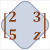Games
Problems
Go Pro!

# Matrices

Reference > Mathematics > AlgebraA brief explanation of matrices - what they areAdding Matrices and Subtracting Matrices - how to perform simple matrix operationsHow to multiply a matrix by a scalar (numerical) valueHow to multiply one matrix by another matrixAn explanation of the multiplicative identity matrix and finding matrix inversesLike us on Facebook to get updates about new resources